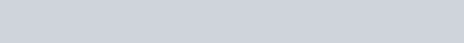A disc revolves with a speed of $33 \frac{1}{3} \mathrm{rev} / \mathrm{min}$,
Question.
A disc revolves with a speed of $33 \frac{1}{3} \mathrm{rev} / \mathrm{min}$, and has a radius of $15 \mathrm{~cm}$. Two coins are placed at $4 \mathrm{~cm}$ and $14 \mathrm{~cm}$ away from the centre of the record. If the co-efficient of friction between the coins and the record is $0.15$, which of the coins will revolve with the record?

solution:

Coin placed at 4 cm from the centre

Mass of each coin = m

Radius of the disc, r = 15 cm = 0.15 m

Frequency of revolution, $v=33 \frac{1}{3} \mathrm{rev} / \mathrm{min}=\frac{100}{3 \times 60}=\frac{5}{9} \mathrm{rev} / \mathrm{s}$

Coefficient of friction, $\mu=0.15$

In the given situation, the coin having a force of friction greater than or equal to the centripetal force provided by the rotation of the disc will revolve with the disc. If this is not the case, then the coin will slip from the disc.

Coin placed at 4 cm:

Radius of revolution, $t^{2}=4 \mathrm{~cm}=0.04 \mathrm{~m}$

Angular frequency, $\omega=2 \pi v=2 \times \frac{22}{7} \times \frac{5}{9}=3.49 \mathrm{~s}^{-1}$

Frictional force, $f=\mu m g=0.15 \times m \times 10=1.5 \mathrm{~m} \mathrm{~N}$

Centripetal force on the coin:

$F_{\text {cent. }}=m r^{\prime} \omega^{2}$

$=m \times 0.04 \times(3.49)^{2}$

$=0.49 \mathrm{~m} \mathrm{~N}$

Since $f>\mathrm{F}_{\text {cent }}$, the coin will revolve along with the record.

Coin placed at 14 cm:

Radius, $r^{\prime \prime}=14 \mathrm{~cm}=0.14 \mathrm{~m}$

Angular frequency, $\omega=3.49 \mathrm{~s}^{-1}$

Frictional force, $f=1.5 \mathrm{~m} \mathrm{~N}$

Centripetal force is given as:

$F_{\text {cent. }}=m r^{\prime \prime} \omega^{2}$

$=m \times 0.14 \times(3.49)^{2}$

$=1.7 \mathrm{mN}$